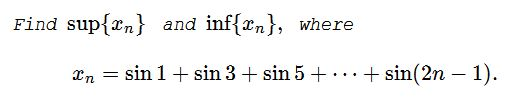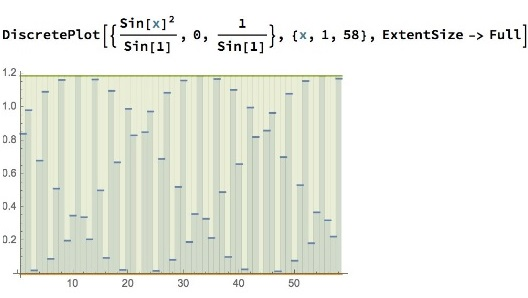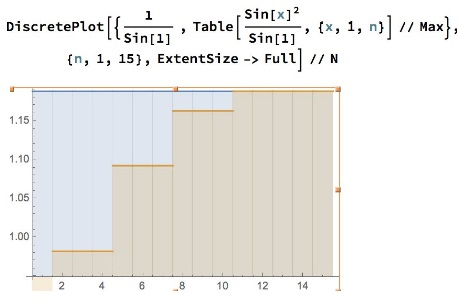### $x_n=\sin 1+\sin 3+\sin 5+\cdots+\sin n$This problem was on the shortlist of the 2014 Olimpiada Nationala de Matematica de Romania and was suggested by Leo Giugiuc. I am grateful to Leo for posting the problem at the CutTheKnotMath facebook page.

### Solution 1

We'll use the following well-known trigonometric formula

$\displaystyle \cos (x-y)-\cos (x+y)=2\sin x \sin y,$

with $y=1:$

\displaystyle\begin{align}\sum_{k=1}^n\sin (2k-1)&=\frac{1}{2\sin 1}\sum_{k=1}^n2\sin (2k-1)\sin 1\\ &=\frac{1}{2\sin 1}\sum_{k=1}^n(\cos (2k-2)-\cos (2k))\\ &=\frac{1}{2\sin 1}(\cos 0-\cos (2n))\\ &=\frac{1-\cos (2n)}{2\sin 1}. \end{align}

Thus $\displaystyle x_n=\frac{1-\cos (2n)}{2\sin 1}\,$ and the problem reduces to finding $\sup\,$ and $\inf\,$ of $\cos (2n).$ Elsewhere we have demonstrated that the values $n\mod\,2\pi\,$ are dense on the unit circle. The same holds for the sequence $2n\mod\,2\pi,\,$ implying that $\inf\{\cos (2n)\}=-1\,$ and $\sup\{\cos (2n)\}=1.\,$ Hence, by noting that $\sin 1\gt 0,\,$, the answers:

$\displaystyle \inf\{x_n\}=\frac{1}{2\sin 1}(1-1)=0\,$ and $\displaystyle \sup\{x_n\}=\frac{1}{2\sin 1}(1+1)=\frac{1}{\sin 1}.$

### Solution 2

This solution in complex numbers is by Mike Lawler.

\displaystyle \begin{align} \sum_{k=1}^n\sin (2k-1) &= \mathbb{Im}\left[\sum_{k=1}^ne^{i(2k-1)}\right]\\ &= \mathbb{Im}\left[\frac{e^{i}(1-e^{2ni})}{1-e^{2i}}\right]\\ &= \mathbb{Im}\left[\frac{e^{i}}{1-e^{2i}}(1-e^{2ni})\right]\\ &= \mathbb{Im}\left[\frac{e^{i}}{1-e^{2i}}(1-\cos 2n+i\sin 2n)\right]\\ &= \mathbb{Im}\left[\frac{i}{2\sin 1}(1-\cos 2n+i\sin 2n)\right]\\ &=\frac{1-\cos 2n}{2\sin 1}, \end{align}

where we used an easily verifiable identity $\displaystyle \frac{e^{i}}{1-e^{2i}}=\frac{i}{2\sin 1}.$ We get the same expression as above and complete the proof with the same density argument.

### Solution 3

The solution is by N. N. Taleb.

As Hadamard observed, the shortest route between two reals is through the complex domain.

From the standard table below, $\sin (x)=\frac{i}{2}(e^{-ix}-e^{ix}),\,$ so $\sin (2k-1)=\frac{i}{2}(e^{-i(2k-1)}-e^{i(2k-1)}).\,$ Now we can use a standard result for the summation of exponential series $\displaystyle \sum_{k=1}^n=\frac{e(e^n-1)}{e-1}.\,$ Accordingly, from the table,

\displaystyle \begin{align} A&=\frac{i}{2}\sum_{k=1}e^{-i(2k-1)}=\frac{i}{2}\frac{e^{i-2in}(-1+e^{2in})}{-1+e^{2i}}\\ &=\frac{i}{2}e^{-in}\frac{\sin (n)}{\sin (1)} \end{align}

and

\displaystyle \begin{align} B&=\frac{i}{2}\sum_{k=1}e^{i(2k-1)}=\frac{i}{2}\frac{e^{i}(-1+e^{2in})}{-1+e^{2i}}\\ &=\frac{-1+e^{2in}}{4\sin (1)} \end{align}

Putting the pieces together, from the table,

\displaystyle \begin{align} x_n&=A-B=\frac{1}{\sin (1)}\left(\frac{i}{2}e^{-in}\sin (n)-\frac{1}{4}(e^{2in}-1)\right)\\ &=\frac{\sin^2 (n)}{\sin (1)}, \end{align}

for, from the same table

$\displaystyle \sin^2(x)=-\frac{1}{4}(e^{-ix}-e^{ix})^2=\frac{1}{2}-\frac{1}{4}e^{-2ix}-\frac{1}{4}e^{2ix}.$

We are dealing with discrete variables, so we do not need to reach the eact supremum for $n=1,2,3\ldots\,$ But the extremum of $\sin (n)\,$ is reached for $n\,$ large and $\sin (x)=\pm 1.\,$ Hence, $\displaystyle \sup x_n=\frac{1}{\sin (1)}\approx 1.1884,\,$ and $\inf x_n=0.$### Telescoping situations

• Leibniz and Pascal Triangles
• Infinite Sums and Products
• Sum of an infinite series
• Harmonic Series And Its Parts
• A Telescoping Series
• An Inequality With an Infinite Series
• That Divergent Harmonic Series
• An Elementary Proof for Euler's Series
• $\sin 1^{\circ}+\sin {2^\circ}+\sin 3^{\circ}+\cdots+\sin 180^{\circ}=\tan 89.5^{\circ}$
• Problem 3824 from Crux Mathematicorum
• $x_n=\sin 1+\sin 3+\sin 5+\cdots+\sin (2n-1)$
• A Welcome Problem for the Year 2018
• ### Related materialRead more...Copyright © 1996-2018 Alexander Bogomolny

 65264597